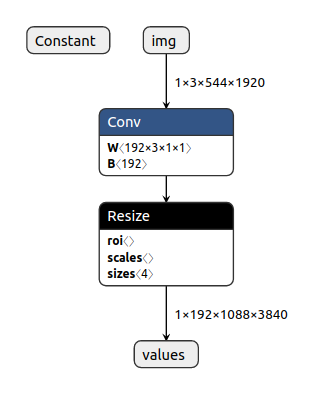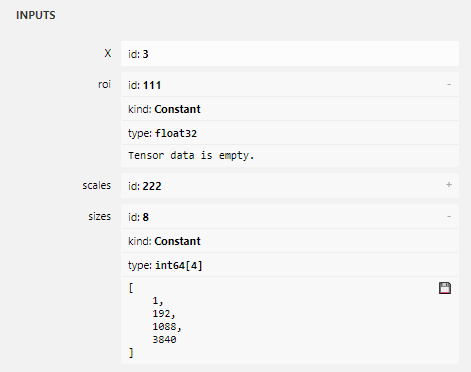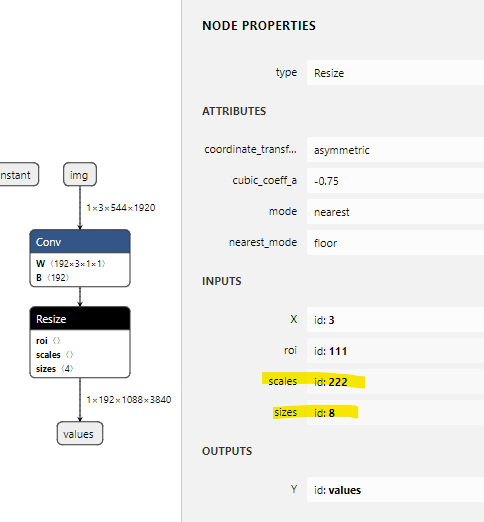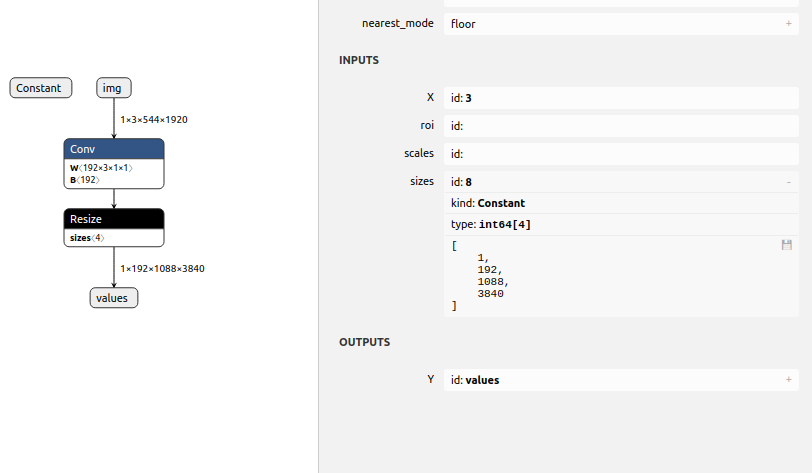# helper.make_tensor_value_info using dynamic variables #2474

Closed
opened this issue Nov 25, 2019 · 14 comments
Closed

# helper.make_tensor_value_info using dynamic variables#2474

opened this issue Nov 25, 2019 · 14 comments

###jinfagang commented Nov 25, 2019

 I am trying to replace a node of a onnx model. but I need calculate the node value: ``````sizes = np.concatenate([part_a, part_b]) # replace resizes input with sizes # new_node = helper.make_tensor_value_info(in_sizes, TensorProto.INT64, sizes) new_node = helper.make_tensor_value_info(in_sizes, TensorProto.INT64, [34, 45, 54]) `````` using static of list can run, but if using value calculated, it throw out error: `````` File "resize_surgery.py", line 52, in get_resize_input_attributes new_node = helper.make_tensor_value_info(in_sizes, TensorProto.INT64, sizes) File "/usr/local/lib/python3.6/dist-packages/onnx/helper.py", line 353, in make_tensor_value_info 'Needs to of integer_types or text_type.'.format(d)) ValueError: Invalid item in shape: 1088. Needs to of integer_types or text_type. ``````

###skottmckay commented Nov 26, 2019

 As the error says the item is not an integer, what types are in part_a and part_b?

###jinfagang commented Nov 26, 2019

 @skottmckay Hi, I am actually doing a thing of this: Resize node exported from torch.onnx.export have a Cast problem when convert to tensorrt using onnx-tensorrt, so I want do some surgery on this node. ``````roi_node = helper.make_node( 'Constant', inputs=['00'], # outputs=['node_resize.input'], outputs=['111'], value=onnx.helper.make_tensor( name='const_tensor', data_type=TensorProto.FLOAT, dims=0, vals=np.array([], dtype=np.float32)) ) scales_node = helper.make_node( 'Constant', inputs=['00'], # outputs=[node_resize.input], outputs=['222'], value=onnx.helper.make_tensor( name='const_tensor', data_type=TensorProto.FLOAT, dims=0, vals=np.array([], dtype=np.float32)) ) sizes_node = helper.make_node( 'Constant', # node name inputs=['00'], # outputs=[in_sizes+'replaced'], outputs=[node_resize.input], value=onnx.helper.make_tensor( name='const_tensor', data_type=TensorProto.INT64, dims=sizes.shape, # dims=4, vals=sizes, ) ) node_resize.input = roi_node.output node_resize.input = scales_node.output node_resize.input = sizes_node.output model.graph.node.append(roi_node) model.graph.node.append(scales_node) model.graph.node.append(sizes_node) `````` Simply, I am just reconstruct this resize node, by calculation `sizes` params and using a Constant feed to it's `sizes` params input. But if I do this, onnx-tensorrt will claim that, the node I added, doesn't have any inputs, it actually does have any inputs, it's just Constant. So does there a way to do it and make it converted to trt engine?

###skottmckay commented Nov 26, 2019

 I still don't see anything showing what's in 'sizes'. Have you tried setting a breakpoint and checking the values to see if they're integers? Regarding the code you pasted, the first argument to helper.make_node is the op type, so you appear to be attempting to create multiple Constant nodes which have inputs. Constant nodes do not have inputs - they have an attribute containing the value to output: https://github.com/onnx/onnx/blob/master/docs/Operators.md#Constant Not sure if it's valid to call make_tensor with the same name 3 different times either.

###jinfagang commented Nov 26, 2019

 I changed name to different name, the error still same, here is my onnx file which generated after surgery: model_upsample_sim.onnx.zip and when call `onnx2trt` , I got: ``````---------------------------------------------------------------- Input filename: model_upsample_sim.onnx ONNX IR version: 0.0.4 Opset version: 11 Producer name: pytorch Producer version: 1.3 Domain: Model version: 0 Doc string: ---------------------------------------------------------------- Parsing model While parsing node number 3 [Resize -> "values"]: ERROR: x/onnx-tensorrt/ModelImporter.cpp:493 In function importModel:  Assertion failed: tensors.count(input_name) `````` As you can see, it claims that my resize's input 111 doesn't have a input tensor. (from source code of onnx-tensorrt I read information about this) Here is my graph:###jinfagang commented Nov 26, 2019

 @skottmckay Could u help me a littele about why? My question is that: why does contant has to have a input, if I want set a constant to a Resize param value, what should I do?

###jinfagang commented Nov 26, 2019

 ``````output: "111" op_type: "Constant" attribute { name: "value" t { data_type: 1 name: "const_tensor1" } type: TENSOR } |raw data that encoded inside this node: []| output: "222" op_type: "Constant" attribute { name: "value" t { data_type: 1 name: "const_tensor2" } type: TENSOR } |raw data that encoded inside this node: []| output: "8" op_type: "Constant" attribute { name: "value" t { dims: 4 data_type: 7 int64_data: 1 int64_data: 192 int64_data: 1088 int64_data: 3840 name: "const_tensor3" } type: TENSOR } `````` It seems it indeed doesn't generates a input field. but this constant is simply constant, why it have to needs a input? How to make it convert to trt-engine?

###skottmckay commented Nov 26, 2019

 Input 111 does not have an input tensor. It's a constant with an empty value. c.f. with 'sizes' which does have values.Additionally the spec for Resize says: Only one of 'scales' and 'sizes' can be specified. scales : tensor(float) The scale array along each dimension. It takes value greater than 0. If it's less than 1, it's sampling down, otherwise, it's upsampling. The number of elements of 'scales' should be the same as the rank of input 'X'. Only one of 'scales' and 'sizes' can be specified. If 'size' is needed, the user can use an empty string as the name of 'scales' in this operator's input list. sizes (optional) : tensor(int64) The size of the output tensor. The number of elements of 'sizes' should be the same as the rank of input 'X'. Only one of 'scales' and 'sizes' can be specified. In your model both scales and sizes are specified. Looks like you're trying to use a constant returning an empty value. As per the spec: If 'size' is needed, the user can use an empty string as the name of 'scales' in this operator's input list.###jinfagang commented Nov 27, 2019

 @skottmckay Thanks for your reply, I think what you said is reasonable. I changed it into empty string now graph looks like this:Here is the new model, however, still, using `onnx2trt` got error: ``````Parsing model node: Resize at input: does have a tensor, will fail. While parsing node number 3 [Resize -> "values"]: ERROR: /onnx2trt_hackbox/onnx-tensorrt/ModelImporter.cpp:493 In function importModel:  Assertion failed: tensors.count(input_name) `````` Any idea?

###skottmckay commented Nov 27, 2019

 Possibly a question for the onnx-tensorrt folks. Looking at their code it's because one of the inputs to a node was not found in the graph inputs, initializers, or output from another node. Can't tell without the onnx file, but it looks like you have a graph input called 'Constant' that isn't connected to anything. Maybe you intended to add a Constant node that produced the output called '8'?

###jinfagang commented Nov 27, 2019

 model_upsample_sim.onnx.zip Here is the onnx model. actually, the Constant outputs 8 is not shown in this graph, it's shown inside Resize. listed all protobuf like this: ``````input: "img" input: "expander.weight" input: "expander.bias" output: "3" op_type: "Conv" attribute { name: "dilations" ints: 1 ints: 1 type: INTS } attribute { name: "group" i: 1 type: INT } attribute { name: "kernel_shape" ints: 1 ints: 1 type: INTS } attribute { name: "pads" ints: 0 ints: 0 ints: 0 ints: 0 type: INTS } attribute { name: "strides" ints: 1 ints: 1 type: INTS } |raw data that encoded inside this node: [1088 3840]| output: "4" op_type: "Constant" attribute { name: "value" t { dims: 2 data_type: 7 raw_data: "@\004\000\000\000\000\000\000\000\017\000\000\000\000\000\000" } type: TENSOR } |raw data that encoded inside this node: []| output: "5" op_type: "Constant" attribute { name: "value" t { dims: 0 data_type: 1 raw_data: "" } type: TENSOR } |raw data that encoded inside this node: []| input: "3" input: "" input: "" input: "8" output: "values" op_type: "Resize" attribute { name: "coordinate_transformation_mode" s: "asymmetric" type: STRING } attribute { name: "cubic_coeff_a" f: -0.75 type: FLOAT } attribute { name: "mode" s: "nearest" type: STRING } attribute { name: "nearest_mode" s: "floor" type: STRING } |raw data that encoded inside this node: []| output: "8" op_type: "Constant" attribute { name: "value" t { dims: 4 data_type: 7 int64_data: 1 int64_data: 192 int64_data: 1088 int64_data: 3840 name: "const_tensor3" } type: TENSOR } ``````

###skottmckay commented Nov 27, 2019

 The graph needs to be topologically sorted. The issue could be that the Constant node producing output "8" is after the Resize node that needs it as input, so it's not available to that node.

###jinfagang commented Nov 27, 2019

 @skottmckay so many thanks for your patience, one last question, how to insert a node before a node? ``````scales_node = helper.make_node( 'Constant', inputs=[], # outputs=[node_resize.input], outputs=['222'], value=onnx.helper.make_tensor( name='const_tensor2', data_type=TensorProto.FLOAT, dims=0, vals=np.array([], dtype=np.float32)) ) sizes_node = helper.make_node( 'Constant', # node name inputs=[], # outputs=[in_sizes+'replaced'], outputs=[node_resize.input], value=onnx.helper.make_tensor( name='const_tensor3', data_type=TensorProto.INT64, dims=sizes.shape, # dims=4, vals=sizes, ) ) # node_resize.input = roi_node.output node_resize.input = '' # node_resize.input = scales_node.output node_resize.input = '' node_resize.input = sizes_node.output # model.graph.node.append(roi_node) # model.graph.node.append(scales_node) model.graph.node.append(sizes_node) `````` I am using this to create and append a node to a graph

###skottmckay commented Nov 27, 2019

 Add the constant nodes (model.graph.node.append) prior to the resize node. Your script doesn't show when you add node_resize, but I assume it happens prior to `model.graph.node.append(sizes_node)`.

###jinfagang commented Nov 28, 2019

 thanks, Resize node is not added, but edit it right on graph. so I have to append it without toopological order. However, this is just out of curiosity but I already found the root reason for this